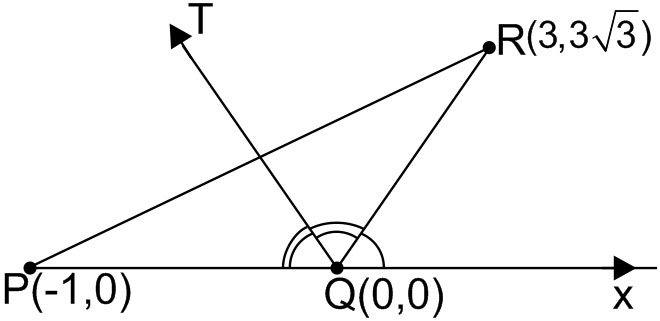Q

# Give answer! - Co-ordinate geometry - JEE Main-6

Let be three points. The equation of the bisector of the angle   is

• Option 1)

• Option 2)

• Option 3)

• Option 4)

120 Views

As learnt in

Slope of a line -

- wherein

Slope of line joining A(x1,y1) and  B(x2,y2) .Slope of line  is

Equation is

Option 1)

This option is incorrect.

Option 2)

This option is incorrect.

Option 3)

This option is correct.

Option 4)

This option is incorrect.

Exams
Articles
Questions